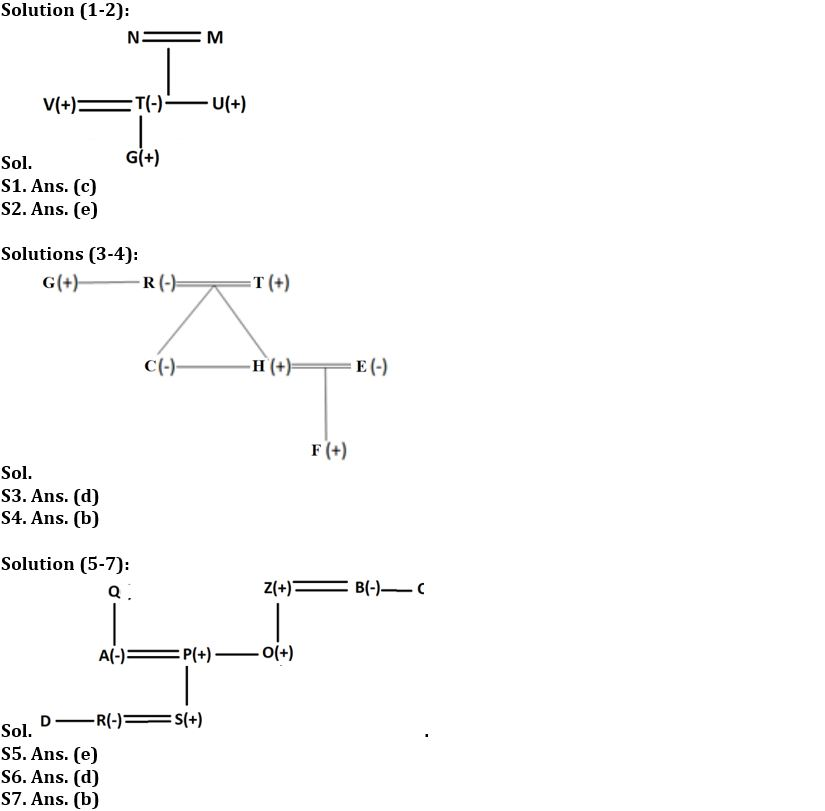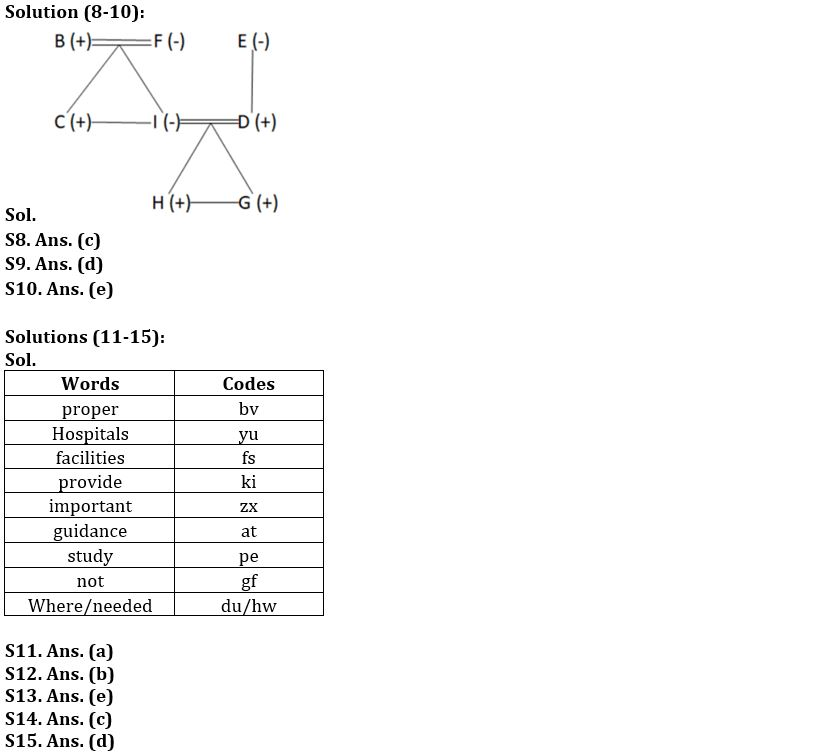Latest Banking jobs   »

# Reasoning Ability Quiz For IBPS SO Prelims 2022- 15th December

Direction (1-2): Read the following information carefully and answer the questions given below.
There is a family consisting of six members named U, M, T, N, G and V. There are two married couples. U is V’s brother-in-law and N’s son. M has only one daughter T who is also U’s sister. N is G’s maternal grandparent. G is male person of the family.

Q1. How is V related to M?
(a) Grandson
(b) Son
(c) Son in law
(d) Husband
(e) None of these

Q2. Who is grandfather of G?
(a) M
(b) N
(c) T
(d) U
(e) Cannot be determined

Direction (3-4): Study the following data carefully and answer the questions accordingly.
R is the mother of C. F is the son of H. G is the brother of R. R has only one daughter. C is the sister of H. T is the father of H. H is married to E.

Q3. How is R related to E?
(a) Sister-in-law
(b) Sister
(c) Mother
(d) Mother-in-law
(e) None of these

Q4. How is H related to R?
(a) Brother
(b) Son
(c) Nephew
(d) Niece
(e) None of these

Direction (5-7): Read the following information carefully and answer the questions given below.
There are ten members in a family of three generations in which there are three married couples. R is the daughter-in-law of P. O who is the sibling of P is the son of Z. C is unmarried. S is the male member of the family. B is the mother of O. Z is the brother-in-law of C.D is the sibling of R. P who is the son-in-law of Q is married to A.

Q5. What is the relation of C with respect to O?
(a) Uncle
(b) Nephew
(c) Niece
(d) Aunt
(e) Either (a) or (d)

Q6. If Q is the father of A, then what is the relation of Q with respect to P?
(a) Father
(b) Mother
(c) Mother-in-law
(d) Father-in-law
(e) None of these

Q7. How many sons do Z has?
(a) One
(b) Two
(c) Three
(d) Cannot be determined
(e) None

Direction (8-10): Study the information carefully and answer the questions accordingly.
B is the father of C who is the brother-in-law of D. F has a son and a daughter. G and H are the grandsons of E. F is the mother-in-law of D. There are at most five males. I is the daughter-in-law of E who has only one child. D is the son of E.

Q8. How many females are there?
(a) Two
(b) Five
(c) Three
(d) Four
(e) None of these

Q9. How is B related to H?
(a) Uncle
(b) Nephew
(c) Son
(d) Grandfather
(e) None of these

Q10. Four from the following five are similar in a certain way and forms a group. Find out the one who does not belong to that group?
(a) H
(b) G
(c) B
(d) C
(e) F

Direction (11-15): Study the following information carefully and answer the questions given below:
In a certain code language:
‘Hospitals provide proper facilities’ is coded as ‘yu ki fs bv’
‘Proper study guidance important’ is coded as ‘bv at zx pe’
‘Hospitals facilities not important’ is coded as ‘zx yu fs gf’
‘facilities guidance where needed’ is coded as ‘fs at hw dn’

Q11. What is the code of “hospital” as per the given code language?
(a) yu
(b) ki
(c) Either (a) or (b)
(d) hw
(e) None of these

Q12. What is the code of “important” as per the given code language?
(a) at
(b) zx
(c) gf
(d) pe
(e) None of these

Q13. What is the code of “guidance needed” as per the given code language?
(a) at pe
(b) at dn
(c) hw at
(d) Either (a) or (b)
(e) Either (b) or (c)

Q14.Which of the following word is coded as ‘bv’ per the given code language?
(a) provide
(b) study
(c) proper
(d) not
(e) None of these

Q15. What may the possible code for ‘provide help’ as per the given code language?
(a) ki bv
(b) bv zx
(c) zx hp
(d) ki hp
(e) ki yu

Solutions#### Congratulations!Union Budget 2023-24: Free PDF